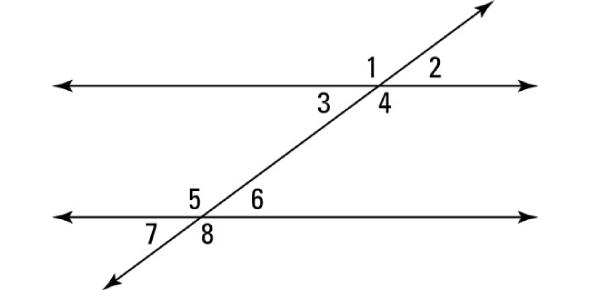# Parallel Lines And Transversals Quiz Questions

10 Questions | Total Attempts: 1778Settings.

• 1.
___________  angles are two angles that are formed by two lines and a transversal and occupy corresponding positions
• 2.
Assume that the two horizontal lines are parallel.  Angles A and B are corresponding angles and therefore not equal.
• A.

True

• B.

False

• C.

• 3.
Which line segment represents the transversal?
• A.

The top parallel line

• B.

The diagonal line intersecting the parallel lines

• C.

The bottom parallel lines

• D.

There are no transversals

• 4.
Alternate exterior angles formed by ________  ________ and a transversal have the same measure.
• 5.
Alternate interior angles formed by parallel lines and a ______________ have the same measure.
• 6.
From the diagram identify which angles are not corresponding angles.
• A.

Angle 4 and Angle 7

• B.

Angle 1 and Angle 3

• C.

Angle 3 and Angle 6

• D.

Angle 5 and Angle 8

• 7.
If lines l an m are parallel, then angles 3 and 4 must be right angles
• A.

True

• B.

False

• 8.
Angle  ___ is one of four acute angles in the illustration
• 9.
Which of the following choices of angles are supplementary to each other?
• A.

1 and 2

• B.

1 and 6

• C.

1 and 8

• D.

1 and 5

• 10.
If angle 1 measures 55 degrees, what does angle 7 equal?
• A.

55

• B.

180

• C.

135

• D.

125

Related TopicsBack to top